• ## 互信息

千次阅读 2017-05-14 20:56:54
香农在信息论中提出了一个“互信息”的概念作为两个随机事件“相关性”的度量。假定有两个随机事件X和Y，它们的互信息定义如下：  I(X;Y)=∑P(x,y)log(P(x,y)/P(x)P(y))   互信息是随机事件X的不确定性或者说熵...
    有关系的信息才能消除不确定性，这个有关系有点模糊，最好能度量“相关性”才好。香农在信息论中提出了一个“互信息”的概念作为两个随机事件“相关性”的度量。假定有两个随机事件X和Y，它们的互信息定义如下：
I(X;Y)=∑P(x,y)log(P(x,y)/P(x)P(y))

互信息是随机事件X的不确定性或者说熵H(X)，以及在知道随机事件Y条件下的不确定性，或者说条件熵H(X|Y)的差异，即I(X;Y)=H(X)-H(X|Y)。现在清楚了，所谓两个事件相关性的量化度量，就是在了解了其中一个Y的前提下，对消除另一个X不确定性所提供的信息量。需要提一下，互信息是一个取值在0到min(H(X),H(Y))之间的函数，当X和Y完全相关时取值为1，当两者完全无关时取值为0。在nlp中，两个随机事件，或者语言特征的互信息是很容易计算的。只要有足够的语料，就不难估计出互信息公式中的P(X,Y)，P(X)和P(Y)三个概率，进而算出互信息。因此互信息被广泛应用于度量一些语言现象的相关性。
机器翻译中，最难的两个问题之一是词义的二义性问题，比如Bush一词可以是美国总统布什的名字也可以是灌木丛。如何正确的翻译呢？人们想到了用语法，分析语句等等。其实迄今为止没有一种语法能很好地解决这个问题，因为Bush不管是翻译成布什还是灌木丛都是名词，在语法上没太大问题。其实真正简单却十分实用的方法是使用互信息。具体解决办法如下：首先从大量文本中找出和总统布什一起出现的互信息最大的词，比如总统、美国，国会等等，再用同样的方法找出和灌木丛一起出现的互信息最大的词，比如土壤、植物、野生等等。有了这两组词，在翻译Bush时，看看上下文哪类相关的词多就可以了。

展开全文nlp 数学
• 参考两个博客 互信息概念与定理 互信息的理解 但第2中公式的变形，实在没看懂，于是推导部分参考wiki，或者这篇
start:
西瓜书7.3
then：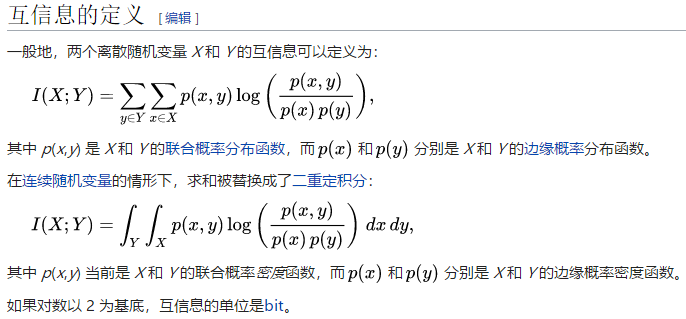参考两个博客
1. 互信息概念与定理
2. 互信息的理解
但第2中公式的变形，实在没看懂，于是推导部分参考wiki，或者这篇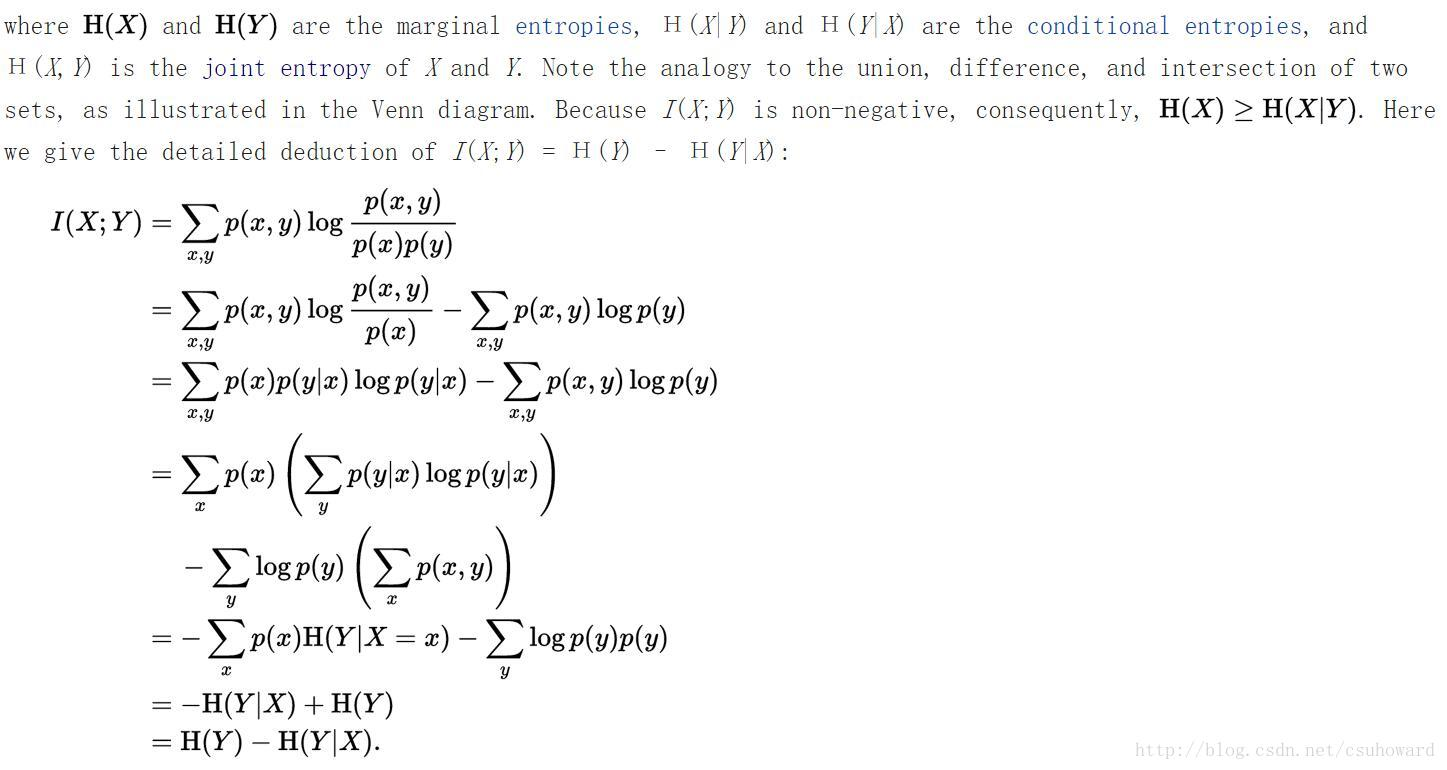参考资料和定义
熵（信息论）
条件熵
互信息


展开全文半朴素贝叶斯
• ## 信息论：熵与互信息

万次阅读 多人点赞 2016-11-25 21:18:43
这篇文章主要讲：熵, 联合熵(joint entropy),条件熵(conditional entropy),相对熵(relative entropy,KL 距离),互信息(mutual information),交叉熵(cross entropy),困惑度(perplexity)。 ...
http://blog.csdn.net/pipisorry/article/details/51695283

这篇文章主要讲：熵, 联合熵(joint entropy),条件熵(conditional entropy),相对熵(relative entropy,KL 距离),互信息(mutual information),交叉熵(cross entropy),困惑度(perplexity)。

熵/信息熵

在信息论中，熵是接收的每条消息中包含的信息的平均量，又被称为信息熵、信源熵、平均自信息量。这里， 消息代表来自分布或数据流中的事件、样本或特征。在信息世界，熵越高，则能传输越多的信息，熵越低，则意味着传输的信息越少。

和热力学熵的联系：在1948年，克劳德·艾尔伍德·香农将热力学的熵，引入到信息论，因此它又被称为香农熵。物理学家和化学家对一个系统自发地从初始状态向前演进过程中，遵循热力学第二定律而发生的熵的变化更感兴趣。在传统热力学中，熵被定义为对系统的宏观测定，并没有涉及概率分布，而概率分布是信息熵的核心定义。

信息熵公式的来源

信息：信息量可以被看成在学习 x 的值的时候的“惊讶程度”。如果有人告诉我们一个相当不可能的时间发生了，我们收到的信息要多于我们被告知某个很可能发生的事件发生时收到的信息，如果我们知道某件事情一定会发生,那么我们就不会接收到信息。于是，我们对于信息内容的度量将依赖于概率分布 p(x) ，因此我们想要寻找一个函数 h(x) ，它是概率 p(x) 的单调递减函数，表达了信息的内容。 h(·) 的形式可以这样寻找：如果我们有两个不相关的事件 x 和 y ,那么我们观察到两个事件同时发生时获得的信息应该等于观察到事件各自发生时获得的信息之和，即 h(x, y) = h(x) + h(y) {采用概率分布的对数作为信息的量度的原因是其可加性。例如，投掷一次硬币提供了1 Sh的信息，而掷 m 次就为 m 位。更一般地，你需要用 log2(n) 位来表示一个可以取 n 个值的变量。}。两个不相关事件是统计独立的，因此 p(x, y) = p(x)p(y) 。根据这两个关系，很容易看出 h(x) 一定与 p(x) 的对数有关，满足这两个条件的函数肯定是负对数形式。因此，我们有

h(x) = − log 2 p(x)                                (1.92)

负号确保了信息一定是正数或者是零。注意，低概率事件 x 对应于高的信息量。

假设一个发送者想传输一个随机变量的值给接收者。这个过程中,他们传输的平均信息量通可以通过求公式(1.92)关于概率分布 p(x) 的期望得到。即事件的概率分布和每个事件的信息量构成了一个随机变量，这个随机变量的均值（即期望）就是这个分布产生的信息量的平均值（即熵）。

熵的定义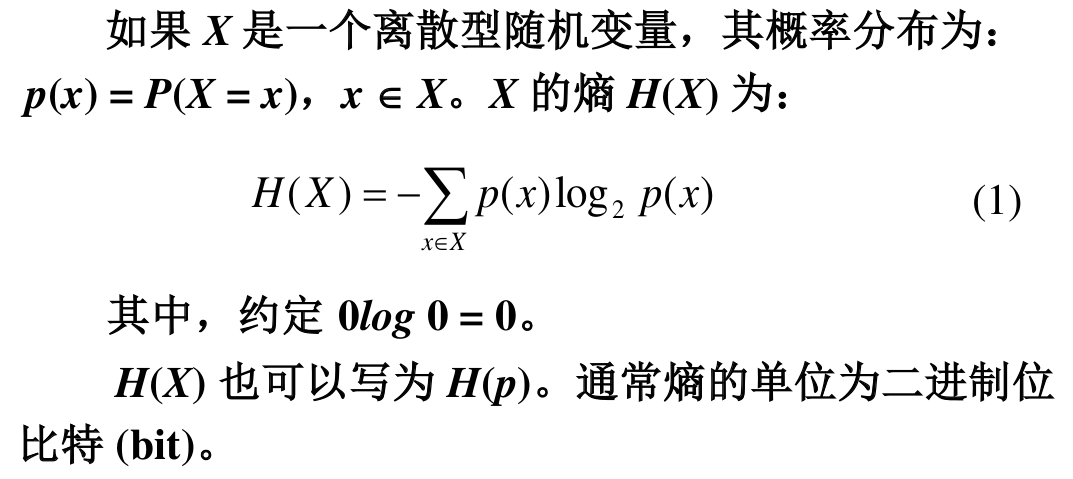依据Boltzmann's H-theorem，香农把随机变量 X 的熵值 Η（希腊字母Eta）定义如下，其值域为 {x1, ...,xn}：$\Eta(X) = \mathrm{E}[\mathrm{I}(X)] = \mathrm{E}[-\ln(\mathrm{P}(X))].$

其中， P 为 X 的概率质量函数（probability mass function），E 为期望函数，而 I(X) 是X 的信息量（又称为自信息）。I(X) 本身是个随机变数。

当取自有限的样本时，熵的公式可以表示为：$\Eta(X) = \sum_{i} {\mathrm{P}(x_i)\,\mathrm{I}(x_i)} = -\sum_{i} {\mathrm{P}(x_i) \log_b \mathrm{P}(x_i)},$

熵又称为自信息(self-information),表示信源 X 每发一个符号(不论发什么符号)所提供的平均信息量。

熵可以理解为不确定性的量度（或者说是多样性diversity的度量），因为越随机的信源的熵越大。熵可以被视为描述一个随机变量的不确定性的数量。一个随机变量的熵越大,它的不确定性越大。那么,正确估计其值的可能性就越小。越不确定的随机变量越需要大的信息量用以确定其值。

熵和最短编码长度的这种关系是一种普遍的情形。无噪声编码定理(oiselesscodingtheorem)(Shannon,1948)表明,熵是传输一个随机变量状态值所需的比特位的下界。

熵的单位

单位取决于定义用到对数的底。当b = 2，熵的单位是bit；当b = e，熵的单位是nat；而当b = 10,熵的单位是 Hart。

熵的取值范围

从定义式,我们可以看出,虽然信息熵经常被称为负熵,但是其取值恒为正,这是因为pi恒小于1。不过，由于信息的接受就是不肯定性的消除，即熵的消除（此处的熵取“热力学熵”中“熵”的含义），所以信息熵才常被人称作负熵。

熵的取值范围为[0~lgK]。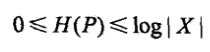其中|X|是X的取值个数。

由于 0 ≤ p i ≤ 1 ,因此熵是非负的。当 p i = 1 且所有其他的 p j̸ = i = 0 时,熵取得最小值0。

在概率归一化的限制下,使用拉格朗日乘数法可以找到熵的最大值。因此,我们要最大化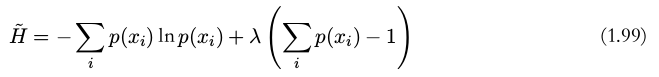可以证明,当所有的 p(x i ) 都相等,且值为 p(x i ) = 1/M 时,熵取得最大值（当且仅当X的分布是均匀分布时右边的等号成立。即当X服从均匀分布时，熵最大）。其中, M 是状态 x i 的总数。此时对应的熵值为 H = ln M 。这个结果也可以通过 Jensen 不等式推导出来。

[Lecture 4 Shannon Information Entropy Maximum and Minimum]

熵的特性

任何满足这些假设的熵的定义均正比以下形式其中，K是与选择的度量单位相对应的一个正比常数。下文中，pi = Pr(X = xi)且连续性

该量度应连续，概率值小幅变化只能引起熵的微小变化。

对称性

符号xi重新排序后，该量度应不变。等。

极值性

当所有符号等可能出现的情况下，熵达到最大值（所有可能的事件等概率时不确定性最高）。。

等概率事件的熵应随符号的数量增加。可加性

熵的量与该过程如何被划分无关。

最后给出的这个函数关系刻画了一个系统与其子系统的熵的关系。如果子系统之间的相互作用是已知的，则可以通过子系统的熵来计算一个系统的熵。

给定n个均匀分布元素的集合，分为k个箱（子系统），每个里面有 b1, ..., bk 个元素，合起来的熵应等于系统的熵与各个箱子的熵的和，每个箱子的权重为在该箱中的概率。

对于正整数bi其中b1 + ... + bk = n来说，。

选取k = n，b1 = ... = bn = 1，这意味着确定符号的熵为零：Η1(1) = 0。这就是说可以用n进制熵来定义n个符号的信源符号集的效率。[信息冗余]

进一步性质

香农熵满足以下性质，借由将熵看成“在揭示随机变量X的值后，从中得到的信息量（或消除的不确定性量）”，可来帮助理解其中一些性质。

增减一概率为零的事件不改变熵：可用琴生不等式证明具有均匀概率分布的信源符号集可以有效地达到最大熵logb(n)：所有可能的事件是等概率的时候，不确定性最大。

计算 (X,Y)得到的熵或信息量（即同时计算X和Y）等于通过进行两个连续实验得到的信息：先计算Y的值，然后在你知道Y的值条件下得出X的值。写作。

如果Y=f(X)，其中f是确定性的，那么Η(f(X)|X) = 0。应用前一公式Η(X, f(X))就会产生所以Η(f(X)) ≤ Η(X)，因此当后者是通过确定性函数传递时，变量的熵只能降低。

如果X和Y是两个独立实验，那么知道Y的值不影响我们对X值的认知（因为两者独立，所以互不影响）：。

两个事件同时发生的熵不大于每个事件单独发生的熵的总和，且仅当两个事件是独立的情况下相等。更具体地说，如果X和Y是同一概率空间的两个随机变量，而 (X,Y)表示它们的笛卡尔积，则。

[熵 (信息论)]

熵的示例

示例1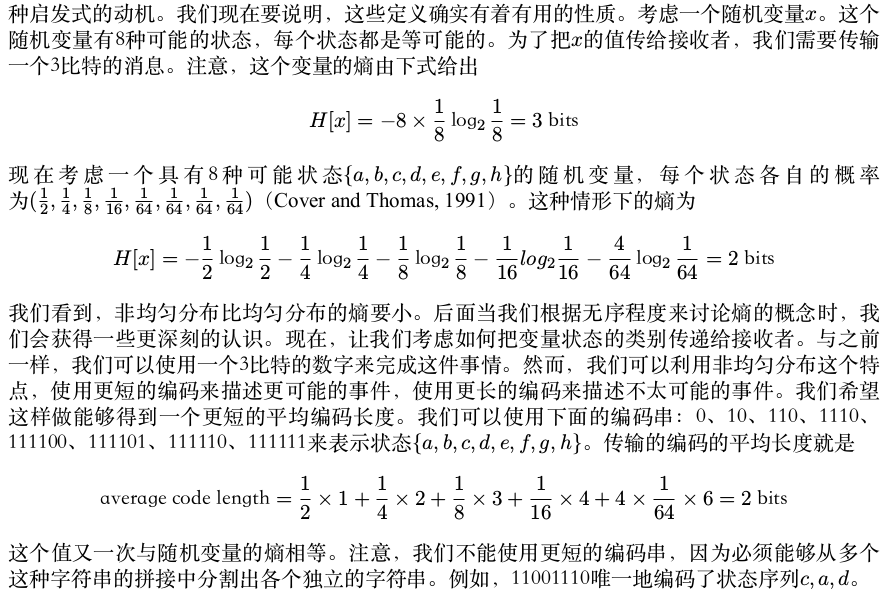至于是如何编码使其平均长度最小的，参考[哈夫曼编码]就知道了。

示例2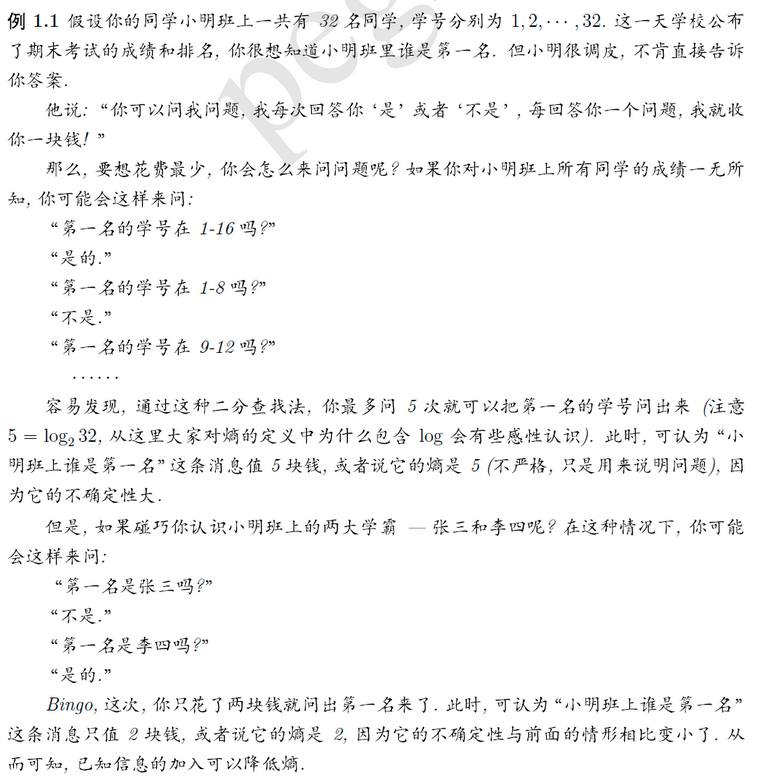皮皮blog

微分熵$\Eta(X) = \sum_{i} {\mathrm{P}(x_i)\,\mathrm{I}(x_i)} = -\sum_{i} {\mathrm{P}(x_i) \log_b \mathrm{P}(x_i)},$

由来

量化连续变量 x :只要 x 落在第 i 个箱子中,我们就把 x 赋值为 x i 。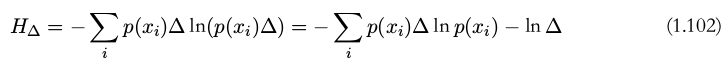我们看到,熵的离散形式与连续形式的差是ln ∆ ,这在极限 ∆ → 0 的情形下发散。这反映出一个事实:具体化一个连续变量需要大量的比特位。

省略公式(1.102)右侧的第二项 − ln ∆ ,然后考虑极限 ∆ → 0 。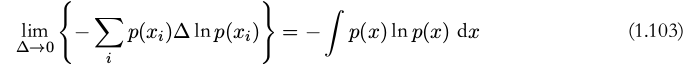微分熵的取值范围

连续变量的最大熵：当一阶和二阶矩受限且保留归一化的限制时，最大化微分熵的分布是高斯分布。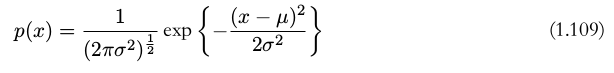Note: 这里熵的单位是nat（底为ln），如果熵的单位是bit（底为lg2），那么最大微分熵会不会是均匀分布？lz没有证实。

可得高斯分布的微分熵：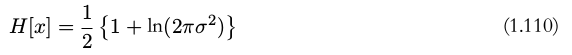因此我们看到熵随着分布宽度(即 σ 2 )的增加而增加。这个结果也表明,与离散熵不同,微分熵可以为负,因为对于公式(1.110),当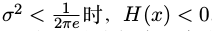。

熵的计算

。。。

给csdn跪了，写的博客自己少了一大半！！！！

香农熵的变型

联合熵(joint entropy)联合熵实际上就是描述一对随机变量平均所需要的信息量。

条件熵(conditional entropy)

可理解为给定X的值前提下随机变量Y的随机性的量。条件熵由来的解释：

假设我们有一个联合概率分布 p(x, y) 。我们从这个概率分布中抽取了一对 x 和 y 。如果 x 的值已知,那么需要确定对应的 y 值所需的附加的信息就是 − ln p(y | x) 。因此,用来确定 y 值的平均附加信息可以写成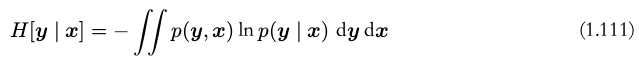因此,描述 x 和 y 所需的信息是描述 x 自己所需的信息,加上给定 x 的情况下具体化 y 所需的额外信息。

皮皮blog

相对熵(relative entropy, 或称 Kullback-Leiblerdivergence, KL 距离)

考虑某个未知的分布 p(x) ,假定我们已经使用一个近似的分布 q(x) 对它进行了建模。如果我们使用 q(x) 来建立一个编码体系,用来把 x 的值传给接收者,那么,由于我们使用了 q(x) 而不是真实分布 p(x) ,因此在具体化 x 的值(假定我们选择了一个高效的编码系统)时,我们需要一些附加的信息。我们需要的平均的附加信息量(单位是 nat )为lz：同时，从这可以看出，只有p(x)的积分是一个定值，所以比较不相似度时也可以去掉第二项，就发现第一项其实就是交叉熵！交叉熵实际上是更广泛的相对熵的特殊情形。注意这个定义前面是没有-号的！！！

可以把 Kullback-Leibler 散度（KL 散度之所以不说距离，是因为不满足对称性和三角形法则）。看做两个分布 p(x) 和 q(x) 之间不相似程度的度量。相对熵常被用以衡量两个随机分布的差距。当两个随机分布相同时,其相对熵为0。当两个随机分布的差别增加时,其相对熵也增加。当q=p时，该度量的结果是0，而其它度量的结果为正值。直观上，它度量了使用q而不是p的压缩损失（以二进制）的程度。假设数据通过未知分布 p(x) 生成,我们想要对 p(x) 建模。我们可以试着使用一些参数分布 q(x | θ) 来近似这个分布。 q(x | θ) 由可调节的参数 θ 控制(例如一个多元高斯分布)。一种确定 θ 的方式是最小化 p(x) 和 q(x | θ) 之间关于 θ 的 Kullback-Leibler 散度。我们不能直接这么做,因为我们不知道 p(x) 。但是,假设我们已经观察到了服从分布 p(x) 的有限数量的训练点 x n ,其中 n = 1, . . . , N 。那么,关于 p(x) 的期望就可以通过这些点的有限加和,使用公式(1.35)来近似,即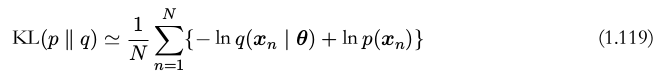公式(1.119)右侧的第二项与 θ 无关,第一项是使用训练集估计的分布 q(x | θ) 下的 θ 的负对数似然函数。因此我们看到,最小化 Kullback-Leibler 散度等价于最大化似然函数。

交叉熵(cross entropy)

如果一个随机变量 X ~ p(x),q(x)为用于近似 p(x)的概率分布,那么,随机变量 X 和模型 q 之间的交叉熵定义为:交叉熵的概念用以衡量估计模型与真实概率分布之间的差异。困惑度(perplexity)

在设计语言模型时,我们通常用困惑度来代替交叉熵衡量语言模型的好坏。PPL是用在自然语言处理领域（NLP）中，衡量语言模型好坏的指标。它主要是根据每个词来估计一句话出现的概率，并用句子长度作normalize，公式为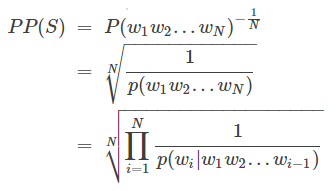或者等价地，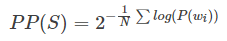即给定语言L的样本语言模型设计的任务就是寻找困惑度最小的模型，使其最接近真实的语言。困惑度越低，说明语言模型面对一句话感到困惑的程度越低，语言模型就越好。说模型的PPL下降到90，可以直观地理解为，在模型生成一句话时下一个词有90个合理选择，可选词数越少，我们大致认为模型越准确。这样也能解释，为什么PPL越小，模型越好。

互信息(mutual information)

如果 (X, Y) ~ p(x, y), X, Y 之间的互信息 I(X; Y)定义为: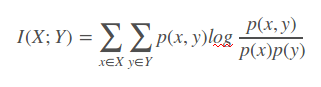Note: 互信息 I (X; Y)取值为非负。当X、Y相互独立时，I(X,Y)最小为0。

互信息实际上是更广泛的相对熵的特殊情形

如果变量不是独立的,那么我们可以通过考察联合概率分布与边缘概率分布乘积之间的 Kullback-Leibler 散度来判断它们是否“接近”于相互独立。此时, Kullback-Leibler 散度为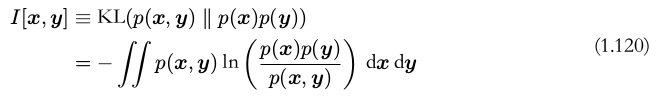这被称为变量 x 和变量 y 之间的互信息( mutual information )。根据 Kullback-Leibler 散度的性质,我们看到 I[x, y] ≥ 0 ,当且仅当 x 和 y 相互独立时等号成立。

使用概率的加和规则和乘积规则,我们看到互信息和条件熵之间的关系为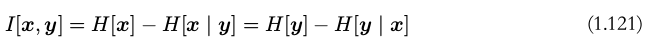可以把互信息看成由于知道 y 值而造成的 x 的不确定性的减小(反之亦然)（即Y的值透露了多少关于X 的信息量）。点互信息PMI（Pointwise Mutual Information）

PMI（Pointwise Mutual Information）这个指标来衡量两个事物之间的相关性（比如两个词）。

其原理很简单，公式如下：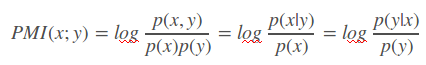在概率论中，我们知道，如果x跟y不相关，则p(x,y)=p(x)p(y)。二者相关性越大，则p(x,y)就相比于p(x)p(y)越大。用后面的式子可能更好理解，在y出现的情况下x出现的条件概率p(x|y)除以x本身出现的概率p(x)，自然就表示x跟y的相关程度。 这里的log来自于信息论的理论，可以简单理解为，当对p(x)取log之后就将一个概率转换为了信息量（要再乘以-1将其变为正数），以2为底时可以简单理解为用多少个bits可以表示这个变量。（更正式的解释请阅读信息论相关理论）

点互信息PMI其实就是从信息论里面的互信息这个概念里面衍生出来的。其衡量的是两个随机变量之间的相关性，即一个随机变量中包含的关于另一个随机变量的信息量。所谓的随机变量，即随机试验结果的量的表示，可以简单理解为按照一个概率分布进行取值的变量，比如随机抽查的一个人的身高就是一个随机变量。可以看出，互信息其实就是对X和Y的所有可能的取值情况的点互信息PMI的加权和，而点互信息只是对其中两个点进行相关性判断。因此，点互信息这个名字还是很形象的。

[点互信息（PMI，Pointwise Mutual Information） ]

互信息、条件熵与联合熵的区别与联系

venn图表示关系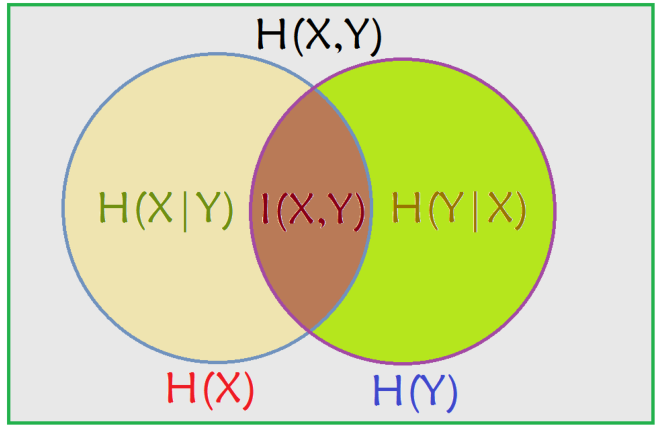由于 H(X|X) = 0, 所以, H(X) = H(X) – H(X|X) = I(X; X)

这一方面说明了为什么熵又称自信息,另一方面说明了两个完全相互依赖的变量之间的互信息并不是一个常量,而是取决于它们的熵。

从图中可以看出，条件熵可以通过联合熵 - 熵（ H(X|Y) = H(X, Y) - H(Y) ）表示，也可以通过熵 - 互信息（ H(X|Y) = H(X) - I(X; Y) ）表示。

皮皮blog

加权熵

香农的经典信息论“只计字数、不计内容”的信息量计算前提，不考虑人的主体意识和状态差别，忽视了不同受信者的这些差别对信息价值和信息量的根本影响，所以没有涉及信息的本质意义。可认为香农的经典信息论只能算作“信号论”、“通信论”或“符号信息论”，香农的“信息量”充其量只能算作信息的“数据量”。

有人研究发现，同一个随机事件发生，对每一个收信人都有不同的意义。如果每一个收信人对各事件都考虑一个意义的因素，定量地给出该事件的权重，那么香农的信息熵（平均信息量）就应该修正为加权熵。于是有了加权熵的定义：

设一个随机变量X包含了一系列（q个）随机事件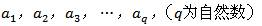（1）

对于某收信人来说，每个事件权重相应的为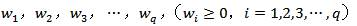（2）

如果事先知道每个事件发生的概率依次为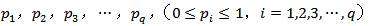（3）

且这些概率满足完备条件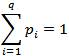（4）

即所有可能的事件发生的概率总和为1，则这个随机变量X可用下述的概率空间来描述：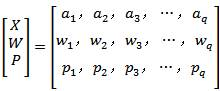（5）

那么，加权熵定义为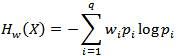（6）

特殊地，如果收信人对信源发生的每一个事件都不感兴趣，或没有意义，也就是说在收信人看来各个事件的权重都为0，即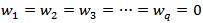（7）

那么即使香农信息熵不为0，按照加权熵定义式（6）计算出来的意义信息量也为0。这就是我们日常生活中司空见惯的“垃圾信息”！

可见，意义信息的加权熵考虑了收信人的关注程度和事件发生对收信人的重要程度，计算出来的平均信息量与香农的信息熵相比更接近实际了一步。

但实际生活中信息的价值和效用不是仅仅用一个权重就可以描述清楚的，况且每个事件的权重也不是都可以用一个数字来定量描述的。所以采用加权熵来描述信息量的作法仍然有其局限性。

[意义信息与加权熵]

雷尼熵/瑞利熵/莱利熵/Renyi熵 Renyi entropy

在信息论中，Renyi系列熵包括香农熵，Hartley熵，最小熵和冲突熵。熵量化了一个系统的多样性，不确定性或者随机性。Renyi熵由Alfred Renyi的名字命名。香农熵只是Renyi熵系列中的一种熵。Renyi熵是对通常的香农熵的扩展，算是q阶广义熵。

设有一离散变量的概率分布(p1,p2,...,pn)，Renyi信息熵定义为：$R(q)=\frac{\log(\sum_{i=1}^{n}p_i^q)}{1-q}$

其中，q为一个可取任意实数的一个参数。

当q=0的时候，R(q)=log(n)，即计算出了元素的个数的对数。

当q=1的时候，分子和分母同时趋近于0，于是，可以通过络必达法则求它的极限为：$\lim_{q\rightarrow 1}R(q)=-\sum_{i=1}^{n}p_i \log(p_i)$

即，当q=1的时候，Renyi熵变成了Shannon信息熵。（证明wiki）

[wikipedia Rényi entropy]
[Renyi熵]

diversity

exp(entropy)

renyi entropy-based diversity:皮皮blog

熵求解示例

熵计算示例1熵计算示例2注意,这里的边缘概率是基于每个音节的,其值是基于每个字符的概率的两倍,因此,每个字符的概率值应该为相应边缘概率的1/2,即:
p: 1/16 t: 3/8 k: 1/16 a: 1/4 i: 1/8 u: 1/8

求联合熵可以有几种方法,以下我们采用连锁规则方法可以得到:皮皮blog

熵的编程实现及拓展包

python实现熵的计算

[Scipy教程 - 统计函数库scipy.stats ]

renyi熵的实现

renyi_entropy_func = lambda pij: 1 / (1 - q) * (np.log2((np.array(pij) ** q).sum()))

[dit/dit: Python package for information theory]

from: http://blog.csdn.net/pipisorry/article/details/51695283

ref: [http://zh.wikipedia.org]

[信息论基础 Tomas Cover]


展开全文条件熵
• 信息熵的相关知识，考虑用信息熵来计算互信息和条件互信息
互信息和条件互信息
最近看了信息熵的相关知识，考虑用信息熵来计算互信息和条件互信息。
MI(X,Y)=H(X)-H(X|Y)
H(X):熵，一种不确定性的度量    H(X,Y):联合熵，两个元素同时发生的不确定度
MI(X,Y)即是信息增益：
H(X,Y)=H(X)+H(Y|X)->H(X|Y)=H(X,Y)-H(Y)
则MI(X,Y)=H(X)+H(Y)-H(X,Y)
MI(X1，X2|Y)=H(X1|Y)-H(X1|X2，Y)=H(X1，Y)-H(Y)-(H(X1，X2，Y)-H(X2，Y)) =H(X1，Y)+H(X2，Y)-H(Y)-H(X1，X2，Y)
正好在R语言当中有直接计算信息熵的包，代码如下：
library(infotheo)

#互信息
mi<-function(mylist1,mylist2){
return(entropy(mylist1)+entropy(mylist2)-entropy(cbind(mylist1,mylist2)))
}
pvalue<-mi(data[,1],data[,2])#测试

#条件互信息
cmi<-function(mylist1,mylist2,mylist3){
return(entropy(cbind(mylist1,mylist3))+entropy(cbind(mylist2,mylist3))-entropy(mylist3)-entropy(cbind(mylist1,mylist2,mylist3)))
}
pvalue1<-cmi(data[,5],data[,13],data[,c(7,8)])

案例结果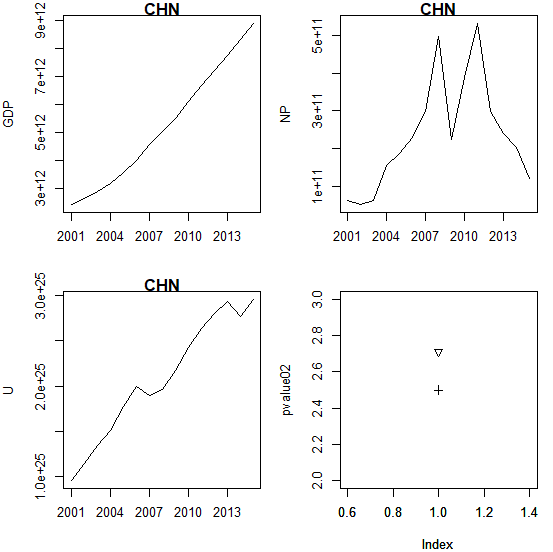setwd("C:\\Users\\Administrator\\Desktop\\MI_cntry 3")
par(mfrow = c(2, 2), mar = c(4, 4, 1, 1))  ###图形分栏布局
#---------------------------------------------------------GDP
str(data1)
zdata1<- t(data1)
mdata1 <- zdata1[-c(1,2),]
m=seq(from = 2001, to = 2015, by=1)  ## 先生成等差数列的刻度，间距为2.5
x1=seq(from =1, to = 15, by=3)
x2=seq(from = 2001, to = 2015, by=3)
plot(mdata1[,1],type='l',main="CHN",xlab="",ylab="GDP",xaxt="n")
axis(side=1,at=x1,labels=x2)

#----------------------------------------------------------NP
str(data2)
zdata2<- t(data2)
mdata2 <- zdata2[-c(1,2),]
m=seq(from = 2001, to = 2015, by=1)  ## 先生成等差数列的刻度，间距为2.5
x1=seq(from =1, to = 15, by=3)
x2=seq(from = 2001, to = 2015, by=3)
plot(mdata2[,1],type='l',main="CHN",xlab="",ylab="NP",xaxt="n")
axis(side=1,at=x1,labels=x2)

#----------------------------------------------------------U

str(data3)
zdata3<- t(data3)
mdata3 <- zdata3[-c(1,2),]
m=seq(from = 2001, to = 2015, by=1)  ## 先生成等差数列的刻度，间距为2.5
x1=seq(from =1, to = 15, by=3)
x2=seq(from = 2001, to = 2015, by=3)
plot(mdata3[,1],type='l',main="CHN",xlab="",ylab="U",xaxt="n")
axis(side=1,at=x1,labels=x2)

#----------------------------------------------------------
############互信息值计算
#install.packages("infotheo")
library(infotheo)
#条件互信息
cmi<-function(mylist1,mylist2,mylist3){
return(entropy(cbind(mylist1,mylist3))+entropy(cbind(mylist2,mylist3))-entropy(mylist3)-entropy(cbind(mylist1,mylist2,mylist3)))
}

#pvalue1<-cmi(mdata1[,1],mdata2[,1],mdata3[,1])

#plot(pvalue1)

#互信息参考网站：https://www.cnblogs.com/jiangyaling/p/8040024.html

mi<-function(mylist1,mylist2){
return(entropy(mylist1)+entropy(mylist2)-entropy(cbind(mylist1,mylist2)))
}
pvalue01<-mi(mdata1[,2],mdata2[,2])#测试
pvalue02<-mi(mdata2[,2],mdata3[,2])#测试

plot(pvalue01,ylin=c(2,3),yaxt="n",ylab="",pch=3)
par(new=TRUE)
plot(pvalue02,ylim=c(2,3),pch=6)



展开全文• 互信息和点互信息 创建日期 星期四 07 三月 2019 点互信息PMI(Pointwise Mutual Information) 点互信息就是很亮两个失误的相关性, 在概率论中，我们知道，如果x跟y不相关，则p(x,y)=p(x)p(y)。二者相关性越大，则...
• ## 互信息介绍

千次阅读 2019-01-05 10:48:05
为什么–为什么要用互信息互信息用在哪里； 是什么–互信息的定义是什么，如何理解； 怎么办–如何进行互信息的计算，具体的示例； 1.2 背景 不知道大家有没有看过《非诚勿扰》这个电视相亲节目？非诚勿扰节目中，...信息增益 特征选择
• 1、点互信息PMI 机器学习相关文献里面，经常会用到点互信息PMI(Pointwise Mutual Information)这个指标来衡量两个事物之间的相关性（比如两个 词）。 其原理很简单，公式如下： 在概率论中，我们知道，如果x跟y不...sklearn
• 之前自己用R写的互信息和条件互信息代码，虽然结果是正确的，但是时间复杂度太高。 最近看了信息熵的相关知识，考虑用信息熵来计算互信息和条件互信息。 MI(X,Y)=H(X)-H(X|Y) H(X):熵，一种不确定性的度量 H(X,Y)...
• 这里给出三个聚类效果评价指标：互信息，标准化互信息，调整互信息(MI, NMI, AMI)，分别给出它们的计算方法与代码。需要指出的是，这三个指标均需要已知数据点的真实标签。 Preliminaries and Notation 已知 NNN 个...聚类 python matlab 机器学习
• 机器学习，信息理论，信息熵的定义、条件熵的定义、联合熵的定义、互信息的定义、条件互信息的定义机器学习
• ## 信息增益与互信息

千次阅读 2018-06-02 17:53:13
信息增益表示得知特征空间X的信息而使得类...特征A对训练数据集D的信息增益 ，定义为集合D的经验熵H(D)与特征A给定条件下D的经验条件熵H(D|A)之差，即通常，熵H(Y)与条件熵H(Y|X)之差称为互信息，决策树学习中的信息...信息增益
• ## 互信息链式法则

千次阅读 2018-04-13 10:11:16
1、2、3、互信息非负。从Y得到X的信息，无关时，互信息最小，为0；
• ## 平均互信息

千次阅读 2016-04-13 14:41:00
集合和事件之间的互信息 定义： 平均互信息：定义 平均互信息I(X;Y)其实就是前面提到的互信息I(x;y)在两个概率空间X和Y中求统计平均的结果，它是从整体上表示一个随机变量Y所给出的关于另一个随机变量X的...
• 在概率论和信息论中，两个随机变量的互信息（Mutual Information，简称MI）或转移信息（transinformation）是变量间相互依赖性的量度。不同于相关系数，互信息并不局限于实值随机变量，它更加一般且决定着联合分布 p...机器学习 MATLAB
• ## 最大互信息系数

千次阅读 2019-12-22 18:54:15
对最大互信息系数的一些思考 最大互信息系数（MIC） MIC（Maximal Information Coefficient）最大互信息系数。用来衡量两个特征变量之间的关联程度（线性或非线性关系），相较于Mutual Information（MI）互信息而言...python 算法
• 研究了下sklearn.feature_selection()中参考的Estimating Mutual Information论文与Mutual Information between Discrete and Continuous Data Sets论文，整理一篇基于k-最近邻的互信息算法。k最近邻
• 互信息互信息互信息PMI 机器学习相关文献里面，经常会用到点互信息PMI(Pointwise Mutual Information)这个指标来衡量两个事物之间的相关性（比如两个词）。 其原理很简单，公式如下： 在概率论中，我们...特征选择
• 本文引自西北工业大学杨宏晖老师的《模式识别之特征选择》 ...识别系统中，特征的信息增益就是一个特征与类别的互信息，该互信息值越大，说明该特征与类别越相关，即该特征包含的分类信息越多，该特征也越重...信息增益
• Excellence is a continuous process and not an ...标准化互信息NMI计算步骤及其Python实现 标准化互信息NMI具体定义可以参考另一篇博客： https://smj2284672469.github.io/2017/10/27/community-detection-meapython
• ## 列向量互信息计算通用MATLAB代码

千次阅读 热门讨论 2018-08-16 17:28:20
互信息的定义 正式地，两个离散随机变量 X 和 Y 的互信息可以定义为： 其中 p(x,y) 是 X 和 Y 的联合概率分布函数，而p(x)和p(y)分别是 X 和 Y 的边缘概率分布函数。 其中 p(x,y) 当前是 X 和 Y 的联合概率密度...机器学习 matlab
• 互信息互信息的话，并没有想象中的那么独立。其实，在互信息的定义中，就是依据自信息来定义的。本质上是通过数学表达式定义的。但是通过这个定义式，不难直接退出一个跟自信息相关的公式。 I(X...math 数学
• 目录一、 计算公式1、信息熵H(X)2、联合熵H(X,Y)3、互信息I(X,Y)4、条件熵H(X|Y)二、举例说明1、信息熵H(X)2、联合熵H(X,Y)3、互信息I(X,Y)4、条件熵H(X|Y)总结 一、 计算公式 在shannon提出信息论到现在已经有70...人工智能 算法
• 我们常用互信息来衡量两个图像的相似程度。互信息的计算方式如下： 使用python中的numpy包或者sklearn可以很方便的计算互信息，计算代码如下： import cv2 import numpy as np import sklearn.metrics as ...python numpy
• ## 互信息图像配准

千次阅读 多人点赞 2015-12-24 22:56:50
图像配准是为了解决两幅不同来源的图像匹配问题。例如，楼外的一颗大树开花了，小明路过觉得好看，就拿相机拍了一张照片。结果回屋拷出来一看不满意，于是小明从窗户...互信息配准算法是解决此问题较好的方法之一。它是图像配准 算法 图像处理
• 在概率论和信息论中，两个随机变量的互信息（Mutual Information，简称MI）或转移信息（transinformation）是变量间相互依赖性的量度。不同于相关系数，互信息并不局限于实值随机变量，它更加一般且决定着联合分布 p...数据挖掘
• MIC 即：Maximal Information Coefficient 最大互信息系数。 使用MIC来衡量两个基因之间的关联程度，线性或非线性关系，相较于Mutual Information（MI）互信息而言有更高的准确度。MIC是一种优秀的数据关联性的计算...机器学习 数据挖掘 特征选择
• 在概率论，尤其是信息论中，条件互信息的基本形式是在给定第三个变量值的情况下，两个随机变量互信息的期望值。 对于离散随机变量X,Y,Z，其条件互信息为： 用图形表示条件互信息为： 具体的定义等后续补充。 ......